### Chemical and Process Engineering Resources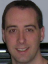## Heat Exchanger Effectiveness

Nov 08 2010 01:40 PM | Chris Haslego in Heat Transfer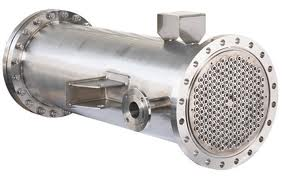Calculating heat exchanger effectiveness allows engineers to predict how a given heat exchanger will perform a new job. Essentially, it helps engineers predict the stream outlet temperatures without a trial-and-error solution that would otherwise be necessary. Heat exchanger effectiveness is defined as the ratio of the actual amount of heat transferred to the maximum possible amount of heat that could be transferred with an infinite area.

Two common methods are used to calculate the effectiveness, equations and graphical. The equations are shown below: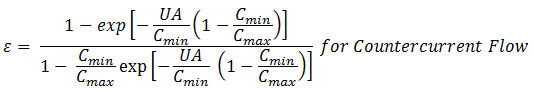Eq. (1)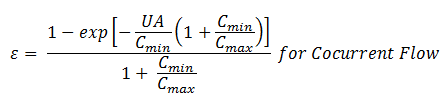Eq. (2)

where:

Â
U = Overall heat transfer coefficient
A = Heat transfer area
Cmin = Lower of the two fluid's heat capacities
Cmax = Higher of the two fluid's heat capacities

Oten times, another variable is defined called the NTU (number of transfer units):
NTU = UA/Cmin

When NTU is placed into the effectiveness equations and they are plotted, you can construct the plots shown below which are more often used than the equations: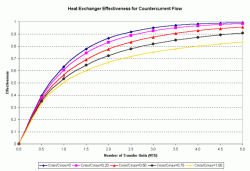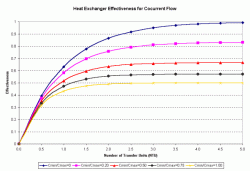Figure 1: Heat Exchanger Effectiveness for Countercurrent Flow Figure 2: Heat Exchanger Effectiveness for Cocurrent Flow
Then, by calculating the Cmin/Cmax and the NTU, the effectiveness can be read from these charts. Once the effectiveness has been found, the heat load is calculated by:

Q = Effectiveness x Cmin x (Hot Temperature in - Cold Temperature in)

and the outlet temperatures can be calculated by:Eq. (3)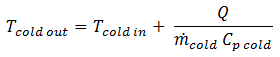Eq. (4)

### Heat Transfer Articles

•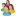Industrial Forum

•Student Forum

•Refining Forum

•Simulation Forum

•Relief Devices Forum

•Tank Venting Forum

•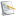Separation Technology

•Calculations and Tips

•Fluid Flow

•Heat Transfer

•Maintenance and Repair

•Utilities

•Safety and Pressure Relief

•Bulk Solids

•Process and Reactions

•Energy

•Other Topics

•For Students

•Physical Properties

•Archived Articles